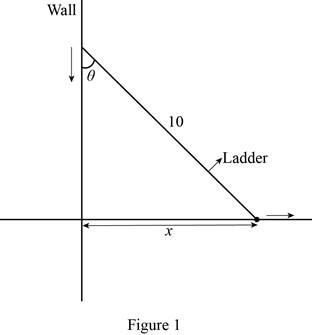# The rate of change of x with respect to θ when θ = π 3 . That is, d x d θ | θ = π 3 .### Single Variable Calculus: Concepts...

4th Edition
James Stewart
Publisher: Cengage Learning
ISBN: 9781337687805### Single Variable Calculus: Concepts...

4th Edition
James Stewart
Publisher: Cengage Learning
ISBN: 9781337687805

#### Solutions

Chapter 3.3, Problem 37E
To determine

## To find: The rate of change of x with respect to θ when θ=π3. That is, dxdθ|θ=π3.

Expert Solution

The rate of change of x with respect to θ when θ=π3 is 5 ft/rad.

### Explanation of Solution

Given:

The length of the ladder is 10 ft, which rests against the vertical wall.

The angle between the top of the ladder and the wall is θ.

The distance from the bottom of the ladder to the wall is x.

Derivative rule:

Constant Multiple Rule:

If c is constant and f(θ). is differentiable function, then

ddθ[cf(θ)]=cddθ[f(θ)] (1)

Calculation:

The given situation is as shown in the below Figure 1.In Figure 1, θ is the angle between the top of the ladder and x is the distance from the bottom of the ladder to the wall.

sinθ=Opposite sideHypotenuse side=x10

Cross multiply the equation,

x=10sinθ

Differentiate the equation with respect to θ,

dxdθ=ddθ(10sinθ)

Apply the Constant Multiple Rule as shown in equation (1),

dxdθ=10ddθ(sinθ)=10cosθ

The rate of change of x with respect to θ when θ=π3 is computed as follows,

dxdθ|x=π3=10(cosπ3)=10(12)      (Q cosπ3=12 )=5

Therefore, the rate of change of x with respect to θ when θ=π3 is 5 ft/rad.

### Have a homework question?

Subscribe to bartleby learn! Ask subject matter experts 30 homework questions each month. Plus, you’ll have access to millions of step-by-step textbook answers!